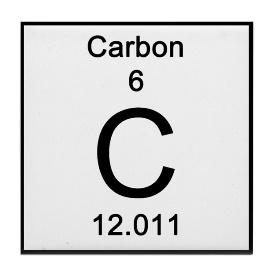# 41.2: Introduction

$$\newcommand{\vecs}{\overset { \rightharpoonup} {\mathbf{#1}} }$$ $$\newcommand{\vecd}{\overset{-\!-\!\rightharpoonup}{\vphantom{a}\smash {#1}}}$$$$\newcommand{\id}{\mathrm{id}}$$ $$\newcommand{\Span}{\mathrm{span}}$$ $$\newcommand{\kernel}{\mathrm{null}\,}$$ $$\newcommand{\range}{\mathrm{range}\,}$$ $$\newcommand{\RealPart}{\mathrm{Re}}$$ $$\newcommand{\ImaginaryPart}{\mathrm{Im}}$$ $$\newcommand{\Argument}{\mathrm{Arg}}$$ $$\newcommand{\norm}{\| #1 \|}$$ $$\newcommand{\inner}{\langle #1, #2 \rangle}$$ $$\newcommand{\Span}{\mathrm{span}}$$ $$\newcommand{\id}{\mathrm{id}}$$ $$\newcommand{\Span}{\mathrm{span}}$$ $$\newcommand{\kernel}{\mathrm{null}\,}$$ $$\newcommand{\range}{\mathrm{range}\,}$$ $$\newcommand{\RealPart}{\mathrm{Re}}$$ $$\newcommand{\ImaginaryPart}{\mathrm{Im}}$$ $$\newcommand{\Argument}{\mathrm{Arg}}$$ $$\newcommand{\norm}{\| #1 \|}$$ $$\newcommand{\inner}{\langle #1, #2 \rangle}$$ $$\newcommand{\Span}{\mathrm{span}}$$$$\newcommand{\AA}{\unicode[.8,0]{x212B}}$$

There is a relationship between moles, grams, and the number of molecules that is similar to the relationship between dozens, grams, and the number of pieces.  One mole of any substance has the same number of particles ($$6 \times 10^{23}$$), just as one dozen of any item has the same number of pieces (12).

Table $$\PageIndex{1}$$: Moles and Molecules

Substance

Molecules

1 mole $$\ce{H2O}$$

$$6 \times 10^{23}$$

1 mole $$\ce{CO}$$

$$6 \times 10^{23}$$

1 mole $$\ce{H}$$

$$6 \times 10^{23}$$

1 mole $$\ce{O3}$$

$$6 \times 10^{23}$$

Item

Pieces

12

1 dozen Roses

12

1 dozen Eggs

12

1 dozen Paperclips

12

The mole is central to stoichiometry like the meter is central to the metric system. If the number of moles are known, then the amount of grams and the number of particles may be calculated.  The atomic mass on the periodic table indicates the amount of grams that one mole of a particular type of atom would contain.  Avogadro’s number is the number of atoms or molecules that 1 mole of any substance would contain.$$N_A =\dfrac{6 \times 10^{23} \text { particles}}{1 \text { mole}}$$ 1 mole of Carbon has 12.011 grams 1 mole of Carbon has $$6 \times 10^{23}$$ atoms